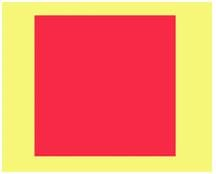A square could also be called a...a)Triangleb...
A square could also be called a...• a)
Triangle
• b)
Circle
• c)
Oblong
• d)
Rectangle
Correct answer is option 'D'. Can you explain this answer?

### Related TestRahul Doiphode May 24, 2020
Related A square could also be called a...a)Triangleb)Circlec)Oblongd)RectangleCorrect answer is option 'D'. Can you explain this answer?
Triangle

 1 Crore+ students have signed up on EduRev. Have you?ANIRUDDH K Apr 29, 2020Rectangles must have 4 sides, four 90 degree angles and two pairs of parallel sidesDurgesh Partani Jun 23, 2020
Related A square could also be called a...a)Triangleb)Circlec)Oblongd)RectangleCorrect answer is option 'D'. Can you explain this answer?
The answer is rectangle shape because four sides , four corners and four angels similar same to square 4⃣.
That's Why.Ananda Kumar Tripathy Jan 03, 2021Rohit Kaushik 2 weeks ago
D partRamesh Ramesh Nov 20, 2021
Related A square could also be called a...a)Triangleb)Circlec)Oblongd)RectangleCorrect answer is option 'D'. Can you explain this answer?
DPrema Rawat Jan 08, 2021
Option BMukul 2arollno22 Nov 24, 2021
RectangleShashi Kant Kant Jan 16, 2021
Related A square could also be called a...a)Triangleb)Circlec)Oblongd)RectangleCorrect answer is option 'D'. Can you explain this answer?
SanjanaYash Mallick Nov 23, 2021
UashChandra Darla Aug 04, 2022
D: rectangle where length and breadths (l=b)are equal for square

Question Description
A square could also be called a...a)Triangleb)Circlec)Oblongd)RectangleCorrect answer is option 'D'. Can you explain this answer? for Class 2 2022 is part of Class 2 preparation. The Question and answers have been prepared according to the Class 2 exam syllabus. Information about A square could also be called a...a)Triangleb)Circlec)Oblongd)RectangleCorrect answer is option 'D'. Can you explain this answer? covers all topics & solutions for Class 2 2022 Exam. Find important definitions, questions, meanings, examples, exercises and tests below for A square could also be called a...a)Triangleb)Circlec)Oblongd)RectangleCorrect answer is option 'D'. Can you explain this answer?.
Solutions for A square could also be called a...a)Triangleb)Circlec)Oblongd)RectangleCorrect answer is option 'D'. Can you explain this answer? in English & in Hindi are available as part of our courses for Class 2. Download more important topics, notes, lectures and mock test series for Class 2 Exam by signing up for free.
Here you can find the meaning of A square could also be called a...a)Triangleb)Circlec)Oblongd)RectangleCorrect answer is option 'D'. Can you explain this answer? defined & explained in the simplest way possible. Besides giving the explanation of A square could also be called a...a)Triangleb)Circlec)Oblongd)RectangleCorrect answer is option 'D'. Can you explain this answer?, a detailed solution for A square could also be called a...a)Triangleb)Circlec)Oblongd)RectangleCorrect answer is option 'D'. Can you explain this answer? has been provided alongside types of A square could also be called a...a)Triangleb)Circlec)Oblongd)RectangleCorrect answer is option 'D'. Can you explain this answer? theory, EduRev gives you an ample number of questions to practice A square could also be called a...a)Triangleb)Circlec)Oblongd)RectangleCorrect answer is option 'D'. Can you explain this answer? tests, examples and also practice Class 2 tests.